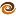# EmptyCrate

#### About Me | Contact Me | Training |ChaiScript.com | YouTube Channel

I recently had a friend point out to me that your typical loop seen in every day code has minor inefficiencies in it which can add up to a good amount of time being wasted. I agreed with him, so I set out to write an article on how to optimize your C++ loops.

The results of the research for this article surprised me. I’ll first cover the optimization techniques:

Typical “Unoptimized” C++ Loop

``````int main()
{
std::vector<uint32_t> vec;

//Fill the vector with some values
for(int i=0; i<10000000; i++)
{
vec.push_back(i);
}

//Sum up the values in the vector 1000 times
for (int i = 0; i < 1000; i++)
{
uint64_t sum = 0;
for (std::vector<uint32_t>::const_iterator itr = vec.begin();
itr != vec.end();
itr++)
{
sum += *itr;
}
std::cout << sum << std::endl;
}
}
``````
 No Optimization 551.97s -O1 48.91s -O2 48.74s

Cached “end()” Iterator

This version of the loop is exactly the same as the unoptimized version, except we are now caching the value of “end()” so that a lookup does not occur on each loop iteration. Not every developer realizes that `for (itr = vec.begin(); itr != vec.end(); itr++)` results in a call to `vec.end()` for each loop. Presumably the compiler cannot cache `.end()` and it may be a very expensive lookup depending on how well or poorly your container is implemented.

``````int main()
{
std::vector<uint32_t> vec;

for(int i=0; i<10000000; i++)
{
vec.push_back(i);
}

for (int i=0; i<1000; i++)
{
uint64_t sum = 0;

//Cache vec.end() to avoid redundant lookups
// (we know that it will not change during this loop but the compiler does not)
std::vector<uint32_t>::const_iterator itr, end(vec.end());
for (itr = vec.begin();
itr != end;
itr++)
{
sum += *itr;
}
std::cout << sum << std::endl;
}
}
``````
 No Optimization 524.81s (5% improvement) -O1 Not Tested -O2 48.78s

We’ve now made a very simple change, we’ve gone from `itr++` to `++itr`. The reason why preincrement is faster than postincrement will be covered in a later article. In this particular case, it saved us almost 40% in our very simple loop!

``````int main()
{
std::vector<uint32_t> vec;

for(int i=0; i<10000000; ++i)
{
vec.push_back(i);
}

for (int i=0; i<1000; ++i)
{
uint64_t sum = 0;
std::vector<uint32_t>::const_iterator itr, end(vec.end());

for (itr = vec.begin();
itr != end;
++itr)
{
sum += *itr;
}
std::cout << sum << std::endl;
}
}
``````
 No Optimization 323.58s (38% Improvement) -O1 Not Tested -O2 48.74s

Use std::for_each

We’ve gone the functional route and put to use `std::for_each` which does the above two things for us, it caches `.end()` and uses pre- instead of post- increment operators for the iterators.

However, in this case, we now see a net loss in effeciency. Why? Because with optimization turned off the compiler is not allowed to inline the calls to `Sum` and `Increment`.

``````struct Sum
{
uint64_t m_sum;

Sum()
: m_sum(0)
{
}

void operator()(uint32_t i)
{
m_sum += i;
}
};

struct Increment
{
int m_value;

Increment(int i)
: m_value(i)
{
}

int operator()()
{
return m_value++;
}
};

int main()
{
std::vector<uint32_t> vec;

//Nice and succinct, use generate_n to generate 10,000,000
//values with the Increment generator
std::generate_n(back_inserter(vec), 10000000, Increment(0));

for (int i = 0; i < 1000; ++i)
{
//Sum and output the values of each vector.
std::cout << std::for_each(vec.begin(), vec.end(), Sum()).m_sum << std::endl;
}
}
``````
 No Optimization 398.65s (23% Loss) -O1 49.95s -O2 48.59s

Technical Conclusion

The preceeding examples and discussion focused on the unoptimized performance characteristics. The surprise? With a modern GCC (these tests were done with GCC 4.2.1) and optimization enabled you cannot affect the performance. At all. Really, anything with -01 or better was within a margin of error, all four examples took approximately 49s each to run, or 85% faster than our fastest un-optimized version.

I should qualify that this conclusion is based on the standard container template classes. It’s possible you. someone on your team or a third party has written rather poor containers with expensive `.end()` lookups and ineffecient iterators such that the techniques above do become more important.

Personal Conclusion

The functional approach is my personal favorite. The code is a tad bit longer, and it is possible that it is slighly slower than the other versions; I did not run enough tests at enough different optimization levels to conclusively determine if it is any slower or faster. However, the code is more reusable: Sum and Increment are generic and could be put in a reusable library, they could even be extended to be templated types to allow you to choose the size of the int you want to support.

Also, the actual loops themselves are much more succicnt and self documenting:

Question: What are you generating?
Answer: An incremented set of integers, starting at 0
Question: What are you doing with the vector?
Answer: Summing all values and outputting the result

The functional programming guys have known this for a long time, C++ is just recently discovering it.

My Videos

Related